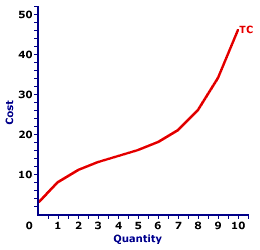Tuesday  June 6, 2023
 AmosWEB means Economics with a Touch of Whimsy!JOB SECURITY: The prospects of continued employment with little or no fear of being forced to leave. Job security is often part of the terms of employment and is designed to reduce uncertainty for both employees and employers. However, it can also reduce worker productivity and restrict the efficient movement of resources between jobs.TOTAL COST CURVE:

A curve that graphically represents the relation between the total cost incurred by a firm in the short-run production of a good or service and the quantity produced. The total cost curve is a cornerstone upon which the analysis of short-run production is built. It combines all opportunity cost of production into a single curve, which can then be used with the total revenue curve to determine profit. The marginal cost curve, THE focal point for the analysis of short-run production, is derived directly from the total cost curve. The shape of the curve reflects increasing marginal returns at small quantities of output and decreasing marginal returns at larger quantities.
The total cost curve graphically represents the relation between total cost and the quantity of production. This curve can be derived in two ways. One is to plot a schedule of numbers relating output quantity and total cost. The other is to vertically add the total variable cost curve and the total fixed cost curve. The slope of the total cost curve is marginal cost. When constructing this curve, it is assumed that total cost changes as a result of changes in the quantity of output produced, while other variables like technology and resource prices are held fixed.

Total Cost CurveThe graph at the right is the total cost curve for the short-run production of Wacky Willy Stuffed Amigos (those cute and cuddly lizards, snakes, armadillos and tarantulas). The quantity of Stuffed Amigos production, measured on the horizontal axis, ranges from 0 to 10 and the total cost incurred in the production of Stuffed Amigos, measured on the vertical axis, ranges from \$3 to \$46.

The most striking feature of the total cost curve is its curvature. The total cost curve emerges from the vertical axis at \$3, then twists and turns its way to \$46. This curve begins relatively steep, then flattens, before turning increasingly steep once again. It is somewhat reminiscent of the total product curve. The reason is that both curves are guided by the law of diminishing marginal returns.

A scenic trip along this curve reveals that the slope of the total cost curve flattens as the first four Stuffed Amigos are produced. This range of output corresponds with increasing marginal returns found in Stage I of production. Increasing marginal returns is what causes the total cost curve to flatten. Continuing this trip shows that the slope of the total cost curve becomes increasingly steeper after the fourth Stuffed Amigo is produced. Steeper and steeper. This range of output corresponds with decreasing marginal returns, and the extremely important law of diminishing marginal returns, found in Stage II of production. Deceasing marginal returns is what causes the total cost curve to become steeper and steeper and steeper.

The total cost curve is frequently used with a total revenue curve to determine the profit maximizing level of production for a firm. However, this curve is perhaps most important as the basis for deriving the average total cost curve and the marginal cost curve. In particular, marginal cost is the slope of the total cost curve.

 <= TOTAL COST AND MARGINAL COST TOTAL COST CURVES =>Recommended Citation:

TOTAL COST CURVE, AmosWEB Encyclonomic WEB*pedia, http://www.AmosWEB.com, AmosWEB LLC, 2000-2023. [Accessed: June 6, 2023].

Check Out These Related Terms...

Or For A Little Background...

And For Further Study...
Search Again?PINK FADFLY[What's This?] Today, you are likely to spend a great deal of time strolling around a discount warehouse buying club hoping to buy either any book written by Isaac Asimov or a how-to book on building remote controlled airplanes. Be on the lookout for neighborhood pets, especially belligerent parrots.Your Complete ScopeThe New York Stock Exchange was established by a group of investors in New York City in 1817 under a buttonwood tree at the end of a little road named Wall Street."I've always believed that if you put in the work, the results will come. I don't do things half-heartedly. Because I know if I do, then I can expect half-hearted results. "-- Michael Jordan, basketball playerMPPMarginal Physical ProductA PEDestrian's Guide Xtra CreditTell us what you think about AmosWEB. Like what you see? Have suggestions for improvements? Let us know. Click the User Feedback link.| | | | | | | | | | |
| | | |

Thanks for visiting AmosWEB